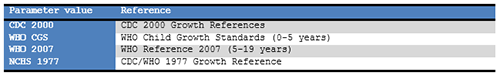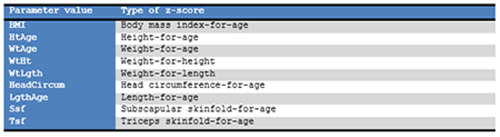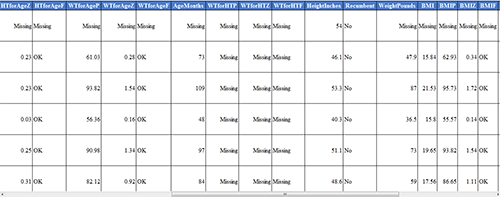# Nutritional Anthropometry: Collecting and Analyzing Nutritional Data

Nutritional Anthropometry

### Using the Nutrition Functions

There may be cases where nutritional data have already been collected in another program (e.g., Microsoft Excel) but the z-scores and percentiles are missing. Re-entering all of this data into the Epi Info™ 7 Nutrition project would be inefficient and time-consuming.

The Epi Info™ 7 Classic Analysis tool contains two specialized functions, ZSCORE and PFROMZ, that can add z-scores and percentiles to an existing set of data. For additional information on the Classic Analysis tool, reference the Classic Analysis section of this user guide.

#### The ZSCORE Function

The ZSCORE function takes a series of parameters and returns a z-score (z statistic) based on those parameters. The nutritional parameters include:

1. Growth reference set (i.e., the CDC 2000 Growth Reference).
2. Type of z-score (i.e., body mass index for age).
3. Name of the column that stores the primary measurement (i.e., BMI).
4. Name of the column that stores the secondary measurement (i.e., AgeMonths).
5. Name of the column that stores the child’s gender, stored as 1’s and 2’s.

Note: The data available in the Nutrition project stores gender information as M and F and must be converted to 1’s and 2’s.

Each parameter is separated by a comma, and the entire series of parameters are enclosed in parenthesis. If you wish to calculate body mass index for age using the CDC 2000 Growth Reference, the function must reference the child’s raw BMI (column BMI), the child’s age in months (column AgeMonths), and the child’s gender (column Gender). The function will be similar to the following:

` ZSCORE("CDC 2000", "BMI", BMI, AgeMonths, Gender).`

The first parameter is “CDC 2000”. The quotes are required as the first two parameters are literal values (i.e. fixed values, the calculations will always refer to the exact CDC 2000 value). The full set of valid references that can be used for this parameter are listed below.Figure 11.12: Valid Values for First Parameter

The second parameter, “BMI”, tells the program to calculate a z-score for body mass index for age. The quotes for this second parameter are also required. The full set of valid z-score types that can be used for the second parameter are listed below. Not all growth references support all types of z-score calculations (i.e., “BMI” may be valid for CDC 2000, but it is not supported when using the NCHS 1977 growth reference).Figure 11.13: Valid Values for Second Parameter

The third parameter is also BMI, but does not contain any quotes because this parameter is the name of the column (or field) in the current dataset that contains the child’s raw BMI calculation. For this example, it is assumed that the raw BMI score is stored in a column called BMI.

The fourth parameter is AgeMonths. It is critical to note that this parameter assumes the specified column is numeric and the numbers represent the child’s age in months.

The fifth parameter is Gender. It assumes the genders are stored in the database as 1’s (male), and 2’s (female).

Note: The data available in the Nutrition project stores gender information as M and F under the field VisitSex. To create the necessary Gender data, enter the following code into Classic Analysis.

```DEFINE Gender NUMERIC
IF VisitSex = "M" THEN
ASSIGN Gender = "1"
ENDIF VisitSex = "F" THEN
ASSIGN Gender = "2"
END```

Database names and column names may differ from the example above. The final three parameters may vary considerably depending on the database. However, the first two parameters will only accept the limited set of inputs stated in the tables above.

To run this calculation, the ZSCORE function must be paired with an ASSIGN command. For example:

` ASSIGN BMIZ = ZSCORE("CDC 2000", "BMI", BMI, AgeMonths, Gender)`Figure 11.14: BMIZ Calculation

The code above instructs the program to assign the z-score for body mass index for age using the CDC 2000 Growth Reference to the BMIZ column. This will be done for every row in the current dataset.

#### PFROMZ Function

The second Nutritional Anthropometry function included in Epi Info™ 7 is called PFROMZ, which converts a z-score into a percentile. To perform the percentile calculation, enter the column name that contains a z-score as a parameter, and the corresponding percentile is returned. For example:

` PFROMZ(BMIZ) `

The above code assumes the BMIZ column contains the z-scores for body mass index for age. Note that the PFROMZ function does not need to know the reference, z-score type, gender, etc., of the data. For example:

` ASSIGN BMIP = PFROMZ(BMIZ) `

The code above instructs the program to take the percentile associated with the z-score values stored in the BMIZ column and to assign that value to the BMIP column. This will be done for every row in the current dataset.

Page last reviewed: September 16, 2022, 12:00 pm